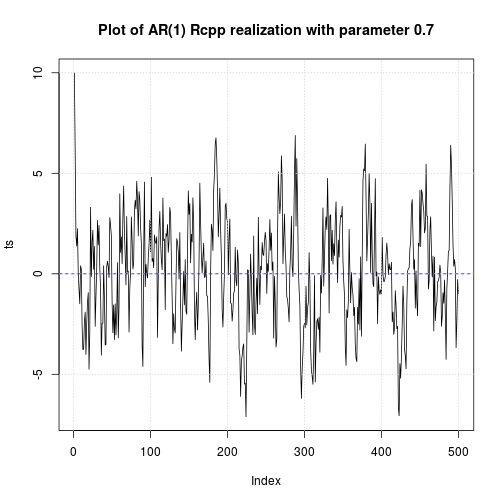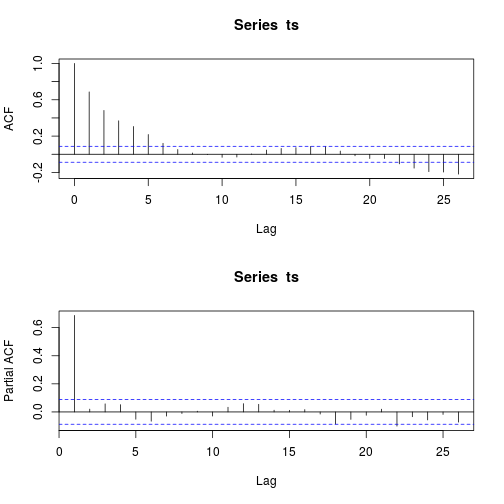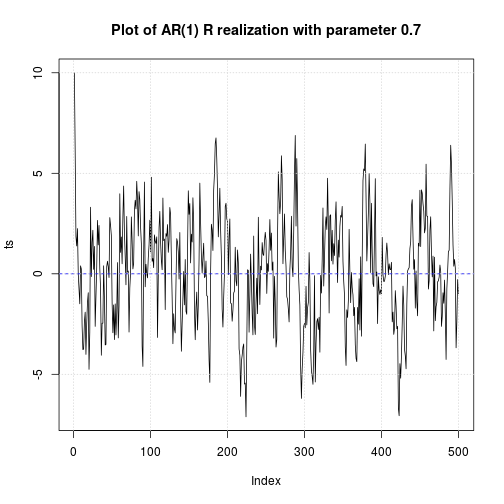Select Page

## Introduction

Sometimes R code just isn’t fast enough and there’s no simply way to make the code any faster.

The Rcpp package provides a consistent API for seamlessly accessing, extending or modifying R objects at the C++ level. The API is a rewritten and extented version of an earlier API which we refer to as the ‘classic Rcpp API’.

## Rcpp, R and C++

All examples in this chapter need at least version 0.10.1 of the Rcpp package. This version includes cppFunction and sourceCpp, which makes it very easy to connect C++ to R. You’ll also need a working C++ compiler.

Before using any of Rccp facilities, we need at least:

Moreover, in order to perfom some comparisons, we also load:

## A simple example with scalars

cppFunction allows developer to write C++ functions in R like this:

Once the function is defined, its print method displays info about the function

and we can use it as any other R function:

Some notes about R and C++ that may be worth to recall before moving forward might be:

• In C++ we must declare the type of output the function returns.
• The classes for the most common types of R vectors are: NumericVector, IntegerVector, CharacterVector and LogicalVector.
• Scalars and vectors in C++ are different.
• The scalar equivalents of numeric, integer, character and logical R vectors are, respectively, double, int, String and bool in C++.

## Brief introduction to vectors

Now let us try a slightly more complex example.

The above function simply returns the sum of elements of a vector passed as a parameter to function sumC().

Note the use of NumericVector to “say” to sumC() that the argument x is a vector containing floating point (double precision) numeric values.

Note also that the C++ code performs an explicit loop on all the vector elements to calculate the sum.

sumC() function is available in .Global environment, as all R functions created by user:

And the speed of sumC() is comparable to speed of native R sum() function:

Now let us try to produce a yet more complex example: a function that calculates the inner product between two equal-length vectors:

We than compare our newly created function with two equivalent R native approaches:

In above example, the function sumP() seems a just about faster than both sum() and the linear algebra-based calculation. Moreover, some small changes to the function may reveal quite sensible advantages either in terms of memory usage, or in terms of performance:

The amount of memory requested by sumP() to perform the computations is close to 0: mmC2[2,6]mmC1[2,6] = 0.1 MB, whereas sum() needed mmR2[2,6]mmR1[2,6] = 763 MB.

This because the calculation performed by sum() is made of two steps:

1. Calculate the vector containing the element by element product
2. Sum the values of products

This produced another side effct: the time requested to perform the inner product alone with sumP() is about 40% of the time requested by sum(), since some time was needed to create the vector and the two calculations “steps” (instead of only one of sumP()) in sum().

The same calculations performed by linear algebra operators return the worst performances both in terms of execution speed and in terms of memory usage.

That is, by a simple function written in C++, we gain sensibily faster and less memory hungry code.

Finally, we could object that the crossprod() R function does the same computations performed by sumP(). This is true, but as an example, this could be equally useful.

Now, let’s try a new problem: we need a function that “generates” (“simulates”) an n-length realization from an AR(1) process with parameter phi, starting from start and having a white noise error with standard deviation equal to sigma.

In this case the return value is not a scalar but a vector. However, the solution is simple:

The arC() function will return a n-length vector directly manageable by R.
Notice in above code the use of rnorm() function, that behave exactly as the R rnorm() function.
In Rcpp sugar (an abstraction layer of Rcpp that provide some facilities to the developer) all the main r/p/q/d R “vector” distribution functions are directly available to programmer.
Notice also the RNGScope scope; line of code, which sets the random number generatator on entry to a block and resets it on exit.

Let’s try the function:Obviously, to avoid many calls to rnorm() in above function, a unique call like rnorm(n,0,sigma) can be made, but this requires more RAM:

Assuming, we want to write a similar function in R, we must write more complicated or much slower code. The simplest, and perhaps slowest, solution is:

And this is the plot:Note that, given the assigned seed, the Rcpp and R functions produce exactly the same numeric output. That means that the compiled C++ code produced by Rcpp is totally integrated with R libraries:

A slightly better R implementation of the same function could be:

The four above functions give the following performance results:

with average performances from 57 to 13 times better for C++ code.

## Using sourceCpp

To simplify the initial presentation, the examples in this chapter have used inline C++ via cppFunction(). For real problems, it’s usually easier to use standalone C++ files and then source them into R using the sourceCpp() function.
This will enable us to take advantage of text editor support for C++ files (e.g. syntax highlighting) as well as make it easier to identify the line numbers of compilation errors.
Standalone C++ files can also contain embedded R code in special C++ comment blocks. This is really convenient if you want to run some R test code.

The standalone C++ file should have extension .cpp, and needs to start with:

And for each function that we want available within R, we need to prefix it with:

where the space after // is mandatory

Then using sourceCpp("path/to/file.cpp") will compile the C++ code, create the matching R functions and add them to current session.
Note that these functions, and the ones created using cppFunction(), will not persist across save() and load(), such as when you restore your workspace.

Let’s try to copy/paste following code into a cppExample1.cpp file in current directory, and compile/run the script as below:

Note the R code block embedded between /*** R and */.
This code will be executed after the compilation to test the functions.

And now meanC() is available in current workspace:

## Matrix computations example

Now we want to write a very simple function that calculates a correlation matrix from a data matrix.
For sake of simplicity, we suppose that the input matrix is numeric and that it does not contain NAs.

In Rcpp, the data types for integer, double, logical, and character matrices are IntegerMatrix, NumericMatrix, LogicalMatrix, CharacterMatrix, respectively.

Of course, the return value of our example function shall be an object of NumericMatrix type.

The above code is saved in cppExample2.cpp source C++ file.
Notice that the R cor() function of stats package has been used within the code. To use the function, an object of class Environment “containing” the stats package has been created, and then from this object a class Function object named corr has been created, where the cor() function of stats package has been assigned.
With these two simple lines of code, the R cor() function has became available to C++ code.
Now we can launch the compilation of code:

And now we can compare the performances of R and Rcpp implementations:

In this specific case, the differences are very small, since the two codes actually implement the same function.

## A last more complex example

The truncgof library contains functions that allow one to calculate GoF statistics, and related p-values, for truncated distributions. The main drawback of these functions is that they use Montecarlo simulations to calculate p-values.
Since the estimates are often obtained by maximization of likelihood, the p-value calculation become a very time-consuming exercize, since for left-truncated distributions, as in this case, the maximum of likelihood is seldom obtainable in closed form, and then it requires a numeric optimization as provided by optim() or similar functions.

We would then like to speed-up the optimization process, and then the p-value calculation too.

Let us try an example: suppose that we have to estimate via MLE the parameters of a left-truncated lognormal distribution. The left truncated distribution function is:

and then the neg-log-likelihood:

The Rcpp code that calculates the truncated distribution and log-likelihood is below, and is saved as cppExample3.cpp file:

Note that two implementations of the same dtrlnorm* procedure have been reported: the first is slightly less performant, but more “concise”, since it uses some facilities of Rcpp Sugar that allows the developer to use vector functions (see the ifelse() function). The only difference between the two nlglik* functions, instead, is on the call to dtrlnormC or dtrlnormC1.

Now let’s compile the above code:

Now, suppose that a sample from a left truncated lognormal distribution has been produced:

We can produce some comparison bechmarks between R and C++ implementations

Note that, at the best, the C++ implementation requires about one-half of time required by the “pure R” code.

An estimate of lognormal parameters may be obtained via maximum likelihood. Below an implementation of a function that performs such as calculation using optim():

Note that the function takes as a parameter the specific instance of neg-log-likelihood function to be used, and that the C++ compiled Rcpp functions can be passed to optim() like all standard R functions.

And now the estimates:

Only very small differences appear between the different implementations.

Now a last benchmark on MLE procedures:

The C++ implementation requires about one half of time required by the R code.

And now, we will use the truncgof package to apply a right-tailed AD2 test on simulated data (see the ad2up.test() help for info about the function):

All the approaches produce the same results, but the best Rcpp implemetation requires a few more than one half of the “pure R” computational time.